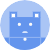search
Search
menu search toc more_vert
Guest 0reps
Thanks for the thanks!
close
account_circle
Profile
exit_to_app
Sign out
help Ask a question
search
keyboard_voice
close
Searching Tips
Search for a recipe:
"Creating a table in MySQL"
Search for an API documentation: "@append"
Search for code: "!dataframe"
Apply a tag filter: "#python"
Useful Shortcuts
/ to open search panel
Esc to close search panel
to navigate between search results
d to clear all current filters
Enter to expand content previewDoc SearchCode Search BetaSORRY NOTHING FOUND!
mic
Start speaking...Voice search is only supported in Safari and Chrome.
Shrink
Navigate to
A
A
brightness_medium
share
arrow_backShareTwitterFacebook
1
thumb_down
0
chat_bubble_outline
0
auto_stories new
settings

# Pandas DataFrame | query method

Pandas
chevron_right
Documentation
chevron_right
DataFrame
chevron_right
Data Selection and Renaming
schedule Jul 1, 2022
Last updated
local_offer PythonPandas
Tags

Pandas' `DataFrame.query(~)` method filters rows according to the provided boolean expression.

NOTE

The `query(~)` method returns a copy of the data, so you cannot use the method to update values of the DataFrame. To perform updates, use properties like `loc` and `iloc`, which returns references instead of copies.

# Parameters

1. `expr` | `string`

The criteria by which to filter the rows. The string must be a boolean expression (e.g. `"A == 2"` and `"A > 3"` where `A` is a column name).

2. `inplace` | `boolean` | `optional`

• If `True`, then the method will directly modify the source DataFrame instead of creating a new DataFrame.

• If `False`, then a new DataFrame will be created and returned.

By default, `inplace=False`.

# Return Value

A `DataFrame` containing the filtered rows.

# Examples

Consider the following DataFrame:

``` import pandas as pddf = pd.DataFrame({"A":[1,2,3], "B":[4,5,6], "C":[7,8,9]})df A B C0 1 4 71 2 5 82 3 6 9 ```

## Equality queries

To get all rows where the value for column `A` is `2`:

``` df.query("A == 2") A B C1 2 5 8 ```

For a not-equals query, use `!=` instead.

## Queries with string values

When referring to string-typed values, wrap them the values in `'` or `"` like so:

``` df = pd.DataFrame({"A":["a","b"]})df.query("A == 'a'") A0 a ```

## Range queries

To get all rows where the value for column `A` is greater than `1`:

``` df.query("A > 1") A B C1 2 5 82 3 6 9 ```

## Interval queries

To get all rows where the value for column `A` is between 1 and 3 (both ends exclusive):

``` df.query("1 < A < 3") A B C1 2 5 8 ```

## AND queries

To get all rows where column `A == 3` and column `C == 9`:

``` df.query("A == 3 and C == 9") A B C2 3 6 9 ```

## OR queries

To get all rows where column `A == 1` or column `C == 9`:

``` df.query("A == 1 or C == 9") A B C0 1 4 72 3 6 9 ```

## Queries with column-comparisons

To get all rows where the value for column `C` is greater than that for column `A`:

``` df.query("C > A") A B C0 1 4 71 2 5 82 3 6 9 ```

## Queries with arithmetic operations

To get all rows where the value for `C` plus 1 is 10:

``` df.query("C + 1 == 10") A B C2 3 6 9 ```

## Queries with object access

You can also access object properties and methods using the dot notation:

``` df.query("B.values.max() < C") A B C0 1 4 71 2 5 82 3 6 9 ```

Here, note the following:

• `B` is of type `Series`, and so you have access to all the properties and methods available for `Series`.

• One such property is `values`, which is simply a `Numpy array` representation of the `Series`.

• Next, we are calling `max()`, which is a method available to all Numpy arrays. In this case, `max()` returns `6` since `6` is the largest value in column `B`.

## IN queries

Consider the following DataFrame:

``` df = pd.DataFrame({"A":["aa","aa","aA","AA"],"B":["b","b","b","b"]})df A B0 aa b1 aa b2 aA b3 AA b ```

To get all rows where the value for `A` is in `arr`:

``` arr = ["aa","AA"]df.query("A in @arr") A B0 aa b1 aa b3 AA b ```

Here, the `arr` is prefixed with `@` since it is an external variable.

## NOT IN queries

Consider the same DataFrame:

``` df = pd.DataFrame({"A":["aa","aa","aA","AA"],"B":["b","b","b","b"]})df A B0 aa b1 aa b2 aA b3 AA b ```

To get all rows where the value for `A` is not in `arr`:

``` arr = ["aa","AA"]df.query("A not in @arr") A B2 aA b ```

## Referencing the index

Consider the following DataFrame:

``` df = pd.DataFrame({"A":[2,3,4],"B":[5,6,7]})df A B0 2 51 3 62 4 7 ```

To refer to the index, simply include the word `index` like so:

``` df.query("index > 1") A B2 4 7 ```

## Handling column names with spaces

When your column names contain spaces, you need to add the back-ticks ``` around the column name.

Consider the following DataFrame:

``` df = pd.DataFrame({"A A":[1,2],"B":[3,4]})df A A B0 1 31 2 4 ```

Here, the first column (i.e. `A A`) contains a space in its name. To deal with this, wrap ``` like follows:

``` df.query("`A A` == 2") A A B1 2 4 ```
mail